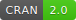`addreg` provides methods for fitting identity-link GLMs and GAMs to discrete data, using EM-type algorithms with more stable convergence properties than standard methods.

An example of periodic non-convergence using `glm` (run with `trace = TRUE` to see deviance at each iteration):

``````require(glm2, quietly = TRUE)
data(crabs)

crabs.boot <- crabs[crabs\$Rep1, -c(5:6)]

t.glm <- system.time(
fit.glm <- glm(Satellites ~ Width + Dark + GoodSpine, data = crabs.boot, family = poisson(identity),
start = rep(1, 4), maxit = 500)
)``````

The combinatorial EM method (Marschner, 2010) provides stable convergence:

``````require(addreg, quietly = TRUE)
t.cem <- system.time(
fit.cem <- addreg(Satellites ~ Width + Dark + GoodSpine, data = crabs.boot, family = poisson,
start = rep(1, 4))
)``````

…but it can take a while. Using an overparameterised EM approach removes the need to run (2^3 = 8) separate EM algorithms:

``t.em <- system.time(fit.em <- update(fit.cem, method = "em"))``

while generic EM acceleration algorithms from the `turboEM` package — implemented in version () 3.0 — can speed this up further still:

``````t.cem.acc <- system.time(fit.cem.acc <- update(fit.cem, accelerate = "squarem"))
t.em.acc <- system.time(fit.em.acc <- update(fit.em, accelerate = "squarem"))``````

Comparison of results:

``````#>         converged    logLik iterations time
#> glm         FALSE -518.2579        500 0.06
#> cem          TRUE -500.8886       6101 0.69
#> em           TRUE -500.8886       1680 0.13
#> cem.acc      TRUE -500.8886        128 0.11
#> em.acc       TRUE -500.8886         38 0.05``````

The combinatorial EM algorithms for identity-link binomial (Donoghoe and Marschner, 2014) and negative binomial (Donoghoe and Marschner, 2016) models are also available, using `family = binomial` and `family = negbin1`, respectively.

Semi-parametric regression using B-splines (Donoghoe and Marschner, 2015) can be incorporated by using the `addreg.smooth` function. See `example(addreg.smooth)` for a simple example.

## Installation

Get the released version from CRAN:

``install.packages("addreg")``

Or the development version from github:

``````# install.packages("devtools")